Courses

# Summary - Resultant of Force System Mechanical Engineering Notes | EduRev

## Mechanical Engineering : Summary - Resultant of Force System Mechanical Engineering Notes | EduRev

The document Summary - Resultant of Force System Mechanical Engineering Notes | EduRev is a part of the Mechanical Engineering Course Engineering Mechanics - Notes, Videos, MCQs & PPTs.
All you need of Mechanical Engineering at this link: Mechanical Engineering

Resultant

Resultant is a single force that will replace a system of forces and produces the same effect on the rigid body as that of the system of forces.

A. RESULTANT OF TWO CONCURRENT FORCES - Parallelogram Law of Forces

 Sign Conventions The following sign conventions shall be used throughout the book1. Upward forces are considered as positive, whereas the downwards as negative.2. Forces acting towards right are considered as positive, whereas those towards left as negative.

PROBLEMS

1. Find the magnitude of the two forces, such that if they act at right angles, their resultant is √10 N. But if they Act at 60°, their resultant is √13 N.

2. The greatest and least resultants of two forces F1 and F2 are 17 N and 3 N respectively. Determine the angles between them when their resultant is √149 N

3. A screw eye is subjected to two forces F1 and F2 as shown in figure. Determine the magnitude and direction of the resultant force by parallelogram method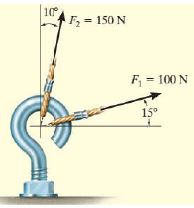4. The two structural members, one of which is in tension and the other in compression, exert the indicated forces on joint O. Determine the magnitude of the resultant R of the two forces and the angle which R makes with the positive x-axis.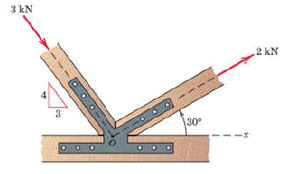Resolution Of Forces

The replacement of a single force by a several components which will be equivalent in action to the given force is called resolution of a force.
Forces can be resolved in any 2 directions. However, it is convenient to resolve them into the two orthogonal components (mutually perpendicular directions)

Resolution of Coplanar Forces in Rectangular Coordinates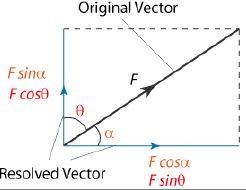B. RESULTANT OF CONCURRENT COPLANAR FORCE SYSTEMSProcedure1. Resolve all the forces into x and y components2. Add the components of forces along the x and y axes with proper sense of direction.3. Find the resultant and inclination of the forces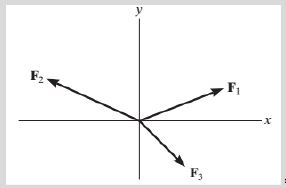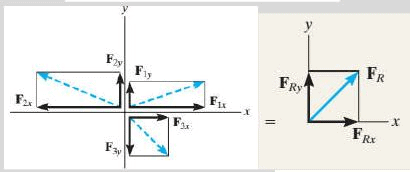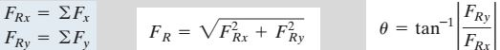Note : 1. If both FRx and FRy are positive, the resultant lies in the first quadrant2. If both FRx and FRy are negative the resultant lies in the third quadrant3. If FRx is positive and FRy is negative, the resultant lies in the fourth quadrant4. If FRx is negative and FRy is positive, the resultant lies in the second quadrant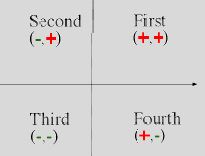PROBLEMS

1. Four forces act on bolt A as shown. Determine the resultant of the force on the bolt.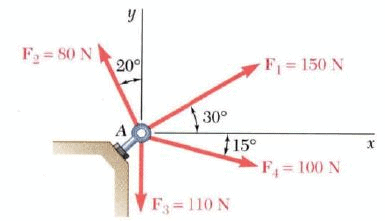2. If the magnitude of the resultant force is to be 9 kN directed along the positive x axis, determine the magnitude of force T acting on the eyebolt and its angle.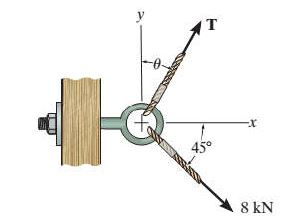3. Determine the resultant of the 3 forces acting on the bracket and its direction.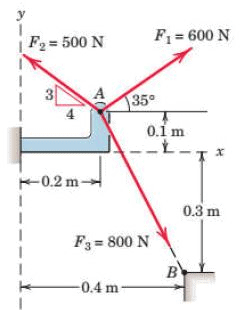4. The forces 20 N, 30 N, 40 N, 50 N and 60 N are acting at one of the angular points of a regular hexagon, towards the other five angular points, taken in order. Find the magnitude and direction of the resultant force.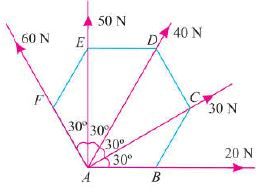5. If Φ = 30 and the resultant force acting on the gusset plate is directed along the positive x axis, determine the magnitudes of F2 and the resultant force.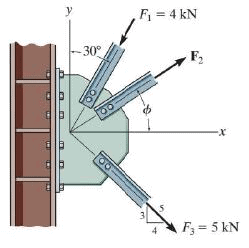Problems for Practice

1. Determine the resultant of the forces shown below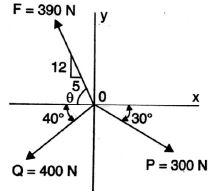2. Determine the resultant of the forces acting on the ring shown in figure.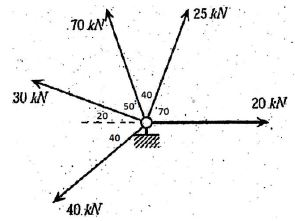3. Find the resultant of the three concurrent forces as shown on figure.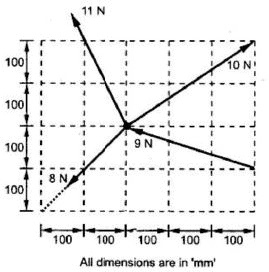4. Find the magnitude and direction of the resultant of the following forces.

i. 20 N inclined at 30° towards North of East.

ii. 25 N towards North.

iii. 30 N towards North West and

iv. 35 N inclined at 40° towards South of West.

Moment

The tendency of a force to rotate the body in the direction of its application a force about a point that is not on the line of action of the force is called Moment of force or simply moment.
Moment is also referred to as torque.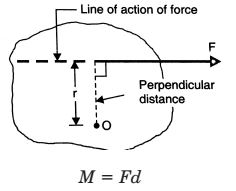Scalar Formulation

Moment is a vector quantity whose direction is perpendicular to the plane of the body. The right-hand rule is used to establish the sense of direction of moment. Throughout the text, clockwise moments are taken as positive while anti-clockwise as negative.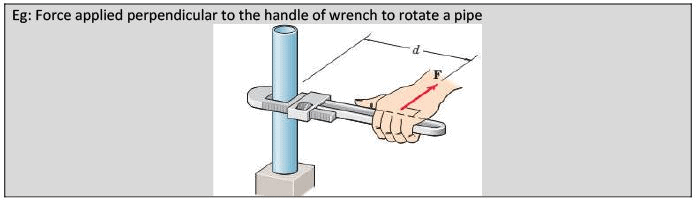Varignon’s Theorem or Principle of Moments

The moment of a force about any point is equal to the sum of the moments of the components of the force about the same point.”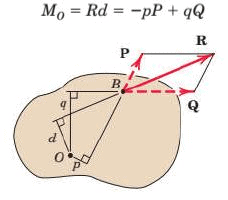Couple

The moment produced by two equal, opposite, and non-collinear forces is called a couple. The perpendicular distance between the lines of action of the two and opposite parallel forces is known as arm of the couple.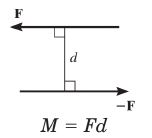A couple can be represented by a vector with magnitude and direction equal to the moment of the couple.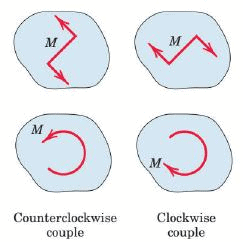Practical Examples : Force apllied to a handle of steering wheel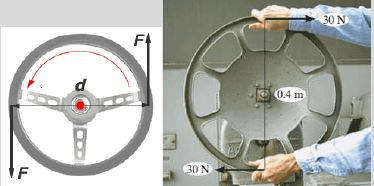Differences between Moment and Couple

 Moment Couple 1 Moment is the tendency of force to rotate a body with the given point or axis2 It is produced by forces not passing through point of rotation axis3 There is a resultant force acting on the body in the direction of force and rotate the body.4 To balance the force causing moment, equal and opposite force is required.5 For example, To tight the nut by spanner To open or close the door 1 Two equal and opposite forces whose lines of action are different form a couple2 It is produced by the two equal and opposite parallel, non collinear forces.3 Resultant force of couple is zero. Hence, body does not move, but rotate only.4 Couple cannot be balanced by a single force, it can be balanced by a couple only.5 For example, To rotate the key in lock To open or close the wheel valve of water line To rotate the steering wheel of car.

C. RESULTANT OF COPLANAR NON-CONCURRENT FORCE SYSTEMS

A system of several forces and couple moments acting on a body can be reduced to equivalent single resultant force acting at a point O and a resultant couple moment.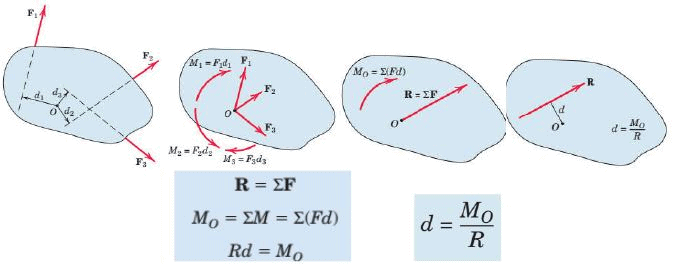X-Y Intercepts Intercepts are the points/coordinates where the line of action meets the corresponding axes.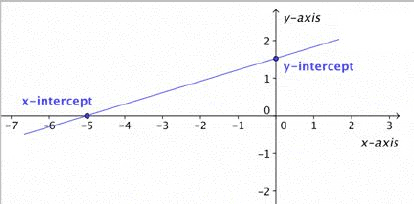D. RESOLUTION OF FORCE INTO FORCE AND COUPLE SYSTEM

Suppose we have to sift he force from point A to B. The procedure to be followed

1. Apply 2 equal and opposite force at point A parallel to force B of the same magnitude

2. If the points are separated by a distance d, the opposite forces F and –F form a couple retaining force F at point B in the same direction as A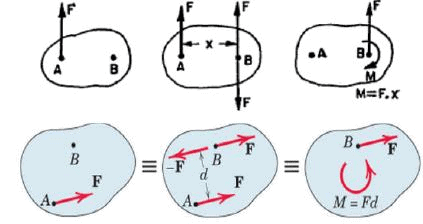PROBLEMS

1. Replace the force system acting on the beam by an equivalent force and couple at point B.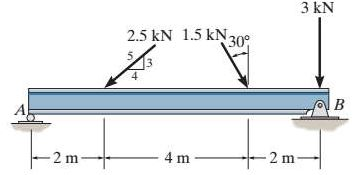2. Reduce the following force system into

a) A single force

b) Resultant force and couple acting at point A.

c) Resultant force and couple acting at point B.

d) Resultant force and couple acting at point C.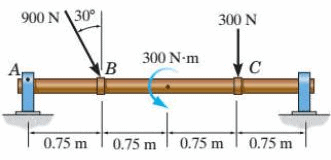3. Replace the force system by a resultant force and couple moment at point O.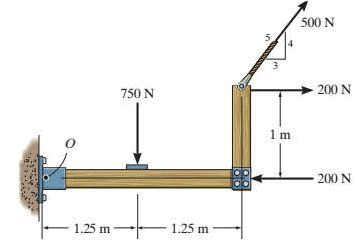4. Two coplanar forces P and Q are shown in figure. Assume all squares of the same size.

i) If P = 4 kN, find the magnitude and direction of Q if their resultant passes through E

ii) If Q = 110 kN, find the magnitude and direction of P if their resultant passes through F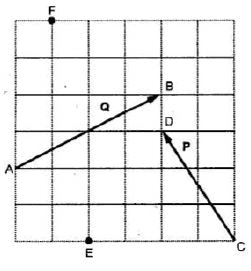Offer running on EduRev: Apply code STAYHOME200 to get INR 200 off on our premium plan EduRev Infinity!

## Engineering Mechanics - Notes, Videos, MCQs & PPTs

30 videos|72 docs|65 tests

,

,

,

,

,

,

,

,

,

,

,

,

,

,

,

,

,

,

,

,

,

;